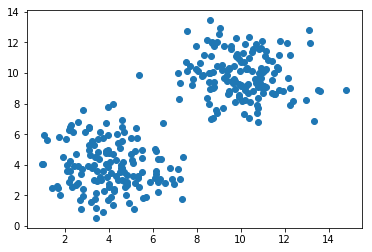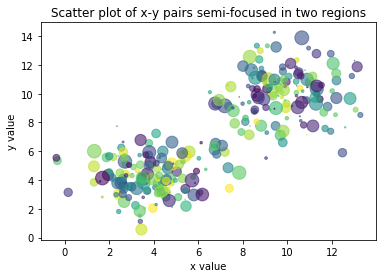Skip to content

# Scatter Plots

## Scatter Plots

Scatter plots of (x,y) point pairs are created with Matplotlib's plt.scatter() function.

The required positional arguments supplied to plt.scatter() are two lists or arrays. The first positional argument specifies the x-value of each point on the scatter plot. The second positional argument specifies the y-value of each point on the scatter plot.

The general form of a plt.scatter() function call shown below.

plt.scatter(x-points, y-points)


The next code section shows how to build a scatter plot with Matplotlib.

First, 150 random, but semi-focused, x and y-values are created using NumPy's np.random.randn() function. The x and y-values are plotted on a scatter plot using Matplotlib's ax.scatter() method. Note the number of x-values is the same as the number of y-values. The size of the two lists or two arrays passed to plt.scatter() must be equal.

In :
import numpy as np
import matplotlib.pyplot as plt
# if uising a Jupyter notebook, include:
%matplotlib inline
x1 = 1.5  np.random.randn(150) + 10
y1 = 1.5  np.random.randn(150) + 10
x2 = 1.5  np.random.randn(150) + 4
y2 = 1.5  np.random.randn(150) + 4
x = np.append(x1,x2)
y = np.append(y1,y2)
fig, ax = plt.subplots()
ax.scatter(x,y)
plt.show()Matplotlib scatter plots can be customized by supplying additional keyword arguments to the ax.scatter() method. Note the keyword arguments used in ax.scatter() are a little different from the keyword arguments used in other Matplotlib plot types.

scatter plot feature ax.scatter() keyword Example
marker size s= ax.scatter(x, y, s=10)
marker color c= ax.scatter(x, y, c=(122, 80, 4))
marker opacity alpha= ax.scatter(x, y, alpha=0.2)

Each of these keyword arguments can be assigned an individual value which applies to the whole scatter plot. The ax.scatter() keyword arguments can also be assigned to lists or arrays. Supplying a list or array controls the properties of each marker in the scatter plot.

The code section below creates a scatter plot with randomly selected colors and areas.

In :
import numpy as np
import matplotlib.pyplot as plt
# if uising a Jupyter notebook, include:
%matplotlib inline
x1 = 1.5  np.random.randn(150) + 10
y1 = 1.5  np.random.randn(150) + 10
x2 = 1.5  np.random.randn(150) + 4
y2 = 1.5  np.random.randn(150) + 4
x = np.append(x1,x2)
y = np.append(y1,y2)
colors = np.random.rand(1502)
area = np.pi  (8  np.random.rand(1502))**2
fig, ax = plt.subplots()
ax.scatter(x, y, s=area, c=colors, alpha=0.6)
ax.set_title('Scatter plot of x-y pairs semi-focused in two regions')
ax.set_xlabel('x value')
ax.set_ylabel('y value')
plt.show()